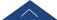﻿ 郑光辉-湖南大学数学学院

# 郑光辉Email

zhenggh2012@hnu.edu.cn

 2012.7-present, Assistant Professor, Hunan University (湖南大学).

 2007.9-2012.7, Ph.D. of applied mathematics, inverse problem for PDE, Lanzhou University (兰州大学：硕博连读).

 2015.3-2016.3, Visting scholar, Ecole Normale Superieure (巴黎高师).

（1）等离子共振分析、隐形设计、超分辨成像

 Z. Q. Miao and G. H. Zheng,  On uniqueness and nonuniqueness for potential reconstruction in quantum fields from one measurement II. the non-radial case, (2019), (Submitted).

 G. H. Zheng and Z. Q. Miao,  On uniqueness and nonuniqueness for potential reconstruction in quantum fields from one measurement, (2019), (Submitted).

 G. H. Zheng,  Mathematical analysis of plasmonic resonance for 2-D photonic crystal,  J. Differential Equations266 (2019), 5095–5117.

（2）贝叶斯统计反问题、偏微分方程反问题

 X. Y. Song, G. H. Zheng and L. J. Jiang,  Variational Bayesian inversion for reaction coefficient in space-time nonlocal diffusion equations, (2019), (Submitted).

 M. H. Ding and G. H. Zheng,  Determination of the reaction coefficient in a time dependent nonlocal diffusion process, (2019), (Submitted).

 G. H. Zheng and M. H. Ding,  Identification of the degradation coefficient for an anomalous diffusion process in hydrology, Inverse Problems (2019) (Accepted).

 G. H. Zheng,  Solving the backward problem in Riesz-Feller fractional diffusion by a new nonlocal regularization method, Appl. Numer. Math., 135 (2019), 99–128.

 X. Y. Song, G. H. Zheng and L. J. Jiang,  Identification of the reaction coefficient in time fractional diffusion equations, J. Comput. Appl. Math.345 (2019), 295–309.

 G. H. Zheng and Q. G. Zhang,  Solving the backward problem for space-fractional diffusion equation by a fractional Tikhonov regularization method, Math. Comput. Simulation, 148 (2018), 37–47.

 G. H. Zheng and Q. G. Zhang, Determining the initial distribution in space-fractional diffusion by a negative exponential regularization method, Inverse Problems in Science and Engineering, (2016).

 G. H. Zheng and Q. G. Zhang, Recovering the initial distribution for space-fractional diffusion equation by a logarithmic regularization method. Appl. Math. Lett. 61 (2016), 143–148.

 G. H. Zheng, Recover the solute concentration from source measurement and

boundary data, Inverse Problems in Science and Engineering, 23 (2015), 1199-1221.

 C. Shi, C. Wang, G. H. Zheng and T. Wei, A new a posteriori parameter

choice strategy for the convolution regularization of the space-fractional backward diffusion problem, Journal of Computational and Applied Mathematics, 279 (2015), 233-248.

 G. H. Zheng and T. Wei, Recover the source and initial value simultaneously

in a parabolic equation, Inverse Problems, 30 (2014), 065013 (35pp).

 H. Cheng, C. L. Fu, G. H. Zheng and J. Gao, A regularization for a Riesz-Feller

space-fractional backward diffusion problem, Inverse Problems in Science and Engineering, 22 (2013), 860-872.

 G. H. Zheng and T. Wei, A new regularization method for a Cauchy problem of the time fractional diffusion equation, Advances in Computational Mathematics, 36 (2012), 377-398.

 G. H. Zheng and T. Wei, A new regularization method for the time fractional

inverse advection-dispersion problem, SIAM Journal on Numerical Analysis, 49 (2011), 1972-1990.

 G. H. Zheng and T. Wei, A new regularization method for solving a time fractional inverse diffusion problem, Journal of Mathematical Analysis and Applications, 378 (2011), 418-431.

 G. H. Zheng and T. Wei, Spectral regularization method for a time fractional

inverse diffusion problem, Applied Mathematics and Computation, 218 (2011), 396-405

 G. H. Zheng and T. Wei, Two regularization methods for solving a Riesz-Feller

space-fractional backward diffusion problem, Inverse Problems, 26 (2010), 115017 (22pp).

 G. H. Zheng and T. Wei, Spectral regularization method for a Cauchy problem

of the time fractional advection-dispersion equation, Journal of Computational and Applied Mathematics, 233 (2010), 2631-2640.

 G. H. Zheng and T. Wei, Spectral regularization method for the time fractional inverse advection-dispersion equation, Mathematics and Computers in Simulation, 81 (2010), 37-51.

(欢迎有一定数学基础，喜欢写程序，或者对概率统计有兴趣的学生报考我的研究生)

Inverse Problems;

Journal of Inverse and Ill-Posed Problems;

Inverse Problems in Science and Engineering;

Journal of Physics A: Mathematical and Theoretical;

Applied Numerical Mathematics;

Mathematical Methods in the Applied Sciences;

Mathematics and Computers in Simulation;

Journal of Engineering Mathematics;

Acta Mathematica Scientia;

 NSF of China (Source identification in spatial domain anomalous diffusion:

regularization theory and algorithms), January, 2014 - December, 2016.

 Funds for the growth of young teachers of Hunan University, September, 2012

- September, 2017.

 Funds for the Ph.D. academic newcomer award of Lanzhou University (Inverse

problems in Fractional PDEs), June, 2011 - June, 2012.

 Numerical Analysis.

 Mathematical Software.

 Numerical solution of PDEs.

  Numerical Analysis. Numerical solution of PDEs. Mathematical Software. Stochastic process.